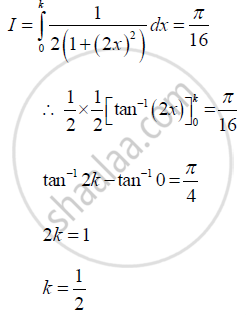# If Integral_0^K 1/(2+ 8x^2) Dx = Pi/16 Then the Value of K is ________. - Mathematics and Statistics

if int_0^k 1/(2+ 8x^2) dx = pi/16 then the value of k is ________.

(A) 1/2

(B) 1/3

(C) 1/4

(D) 1/5

#### Solution

1/2Concept: Definite Integral as the Limit of a Sum
Is there an error in this question or solution?